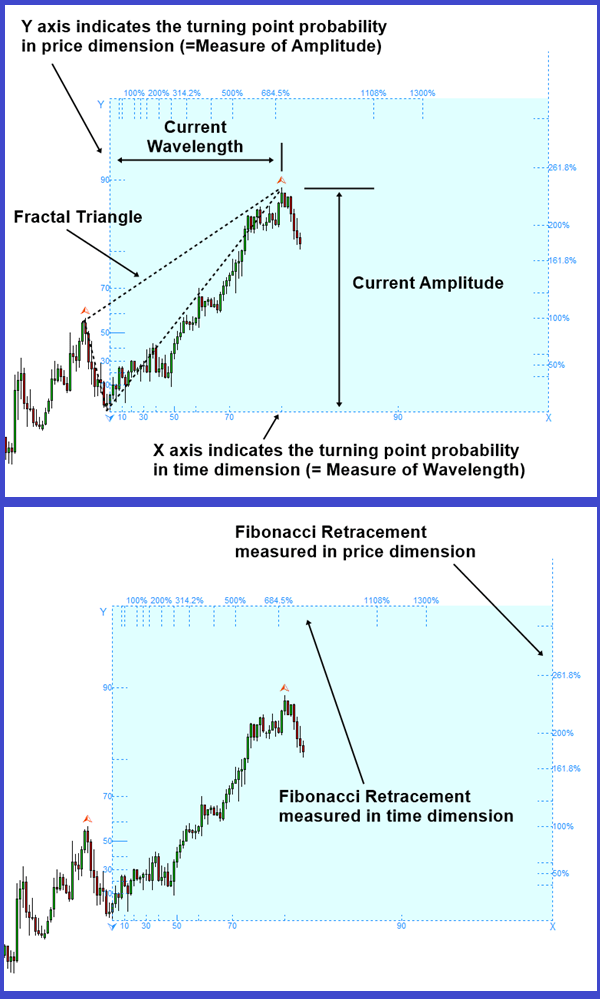# Trend Probability in Forex and Stock market trading

In this article, we will introduce the trend probability for your Forex and Stock market trading. When the turning point probability is high, we can expect the turning point to arrive within the fractal cycle. Like the margin of safety described by Benjamin Graham, it is safe to use the turning point probability in conservative manner. We should only look for the turning point within the higher probability area. The margin of safety will tell that higher turning point probability is safer to predict the turning point. On the other hand, we can look for the breakout opportunity or we can expect the price to move in the same direction within the lower turning point probability area. We can consider the trend probability providing the opposite concept of the turning point probability. In a simple math, we can derive the trend probability by subtracting the turning point probability from one like “1.0 – the turning point probability”. Or if you prefer the percent, we can derive the trend probability by subtracting the turning point probability (%) from one hundred percent like “100% – turning point probability (%)”. Since the turning point probability is drawn from the peak trough transformation directly, the conversion of the trend probability from the turning point probability should be taken as the rule of thumb guideline for your trading. As in the turning point probability, the margin of safety will tell that higher trend probability is safer to predict the breakout or price continuation. This information was taken from the book: Science Of Support, Resistance, Fibonacci Analysis, Harmonic Pattern, Elliott Wave and X3 Chart Pattern (In Forex and Stock Market Trading). This book will provide the scientific rational for chart patterns, connection with economics, chart pattern detection examples, how to use them and how to improve them in Forex and Stock market.

The book can be found in all the major book distributors in the world. Please choose the best book distributor you like from the link below including amazon.com, Google Play Book, scribd.com, Apple Book and so on.

https://www.scribd.com/book/515253555/Science-Of-Support-Resistance-Fibonacci-Analysis-Harmonic-Pattern-Elliott-Wave-and-X3-Chart-Pattern

To present the turning point probability in Forex and Stock Market, we have devised the simple turning point probability graph. When we predict the peak, in the probability graph, Y axis (=the left axis) indicates the turning point probability in price dimension and X axis (= the bottom axis) indicates the turning point probability in time dimension. The probability ranges from 0.0 (=0%) to 1.0 (=100%). Typically, there is no practical application making use of 100% probability in the world. Since our calculation is based on the price data only (i.e. empirical probability), we recommend using 90% probability or less for the maximum probability. For example, even if the price stays above the 90% probability, it is better to treat them as 90% or less depending on how conservative your trading style is.

You have the full access to the turning point probability and trend probability from Fractal Pattern Scanner to improve your Forex and Stock Market Trading. As it was suggested from the book, the display probability in the Fibonacci Probability Graph is the turning point probability. You will use the turning point probability to gauge the trend probability as it was guided from the book. Please have a look at the indicator. Of course, you have this tool built in our Optimum Chart too.

Below is the landing page for Fractal Pattern Scanner in MetaTrader version.

https://www.mql5.com/en/market/product/49170

https://www.mql5.com/en/market/product/49169